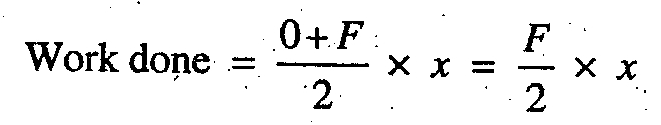# Mechanical Work | Definition | Formula

What is Mechanical Work? Definition and Formula of workdone?

Whenever a force (F) acts on a body and the body undergoes a displacement (x) in the direction of the force, then the work is said to be done. Mathematically, workdone is defined as:If the force varies from 0 to a maximum value of F, then work done is:The unit of work depends upon the unit of force and the displacement. In S.I. system of units, the practical unit of work is N-m. It is the work done by a force of 1 newton when it displaces a body through 1 metre. The work of 1N-m is known as joule (briefly written as J), such that 1 N-m = 1 J.

Note : If a couple or torque (T) acting on a body causes the angular displacement (θ) about an axis perpendicular to the plane of the couple, then,

Workdone = T.θ